# Introduction — What was lacking in the earlier model?

In my previous article, in order to better understand pollution dynamics in Chennai, I used a Gaussian process regression to decompose Chennai’s air pollution data into various sub-components. In this (slightly technical) article, I try to improve the model used in the previous article.

The main issue with the model used earlier was that it assumed that the observations follow a Gaussian distribution. This modelling assumption is not quite aligned with the observed data. The distribution of ‘NowCast’ smoothed PM 2.5 concentration is presented in the figure below.

The distribution is clearly not symmetric about the mean (quite far from it in fact). Hence, assuming the distribution of the outcome variable to be Gaussian would not result in a good fit. However, the distribution of the data does resemble a ‘log-normal’ distribution (i.e., the natural logarithm of hourly PM 2.5 concentration resembles a Gaussian distribution).

There is a slight challenge in transforming the data by taking natural logarithm and then running the Gaussian Process regression, since we have some pesky non-positive values in the data. The natural logarithm is not defined for zero and is complex-valued for negative numbers! To circumvent this issue, I add a small positive value to all observations in the data, such that it shifts the distribution to the right, ensuring that there are no negative values. After shifting the data to the right, we can then take the natural logarithm (logarithm for short). Hence, we have,

where, ‘y hat’ represents the transformed data, from the NowCast PM 2.5 concentration ‘y’. The distribution of this transformed variable (y hat) is closer to a normal distribution (see figure below).

The Gaussian Process regression¹ in the transformed case is the following,

Where the priors are,

The kernel of the Gaussian Process (k(x,x’)) is the squared exponential (SE) kernel², the x_i’s refer to the time when the corresponding observation y_i’s were taken. Time is counted as the number of hours since the midnight of January 1, 2019.

S refers to the vector representing the collection of indicator variables. The model includes an indicator variable for each of the months and special days (the total number of indicator variables is N_s). Beta is a vector with the corresponding coefficients for the indicator variables. As in the previous article, the betas have standard normal priors.

Now, to recover back y, we have,

where, ‘exp’ is the exponential function.

This substantially changes the interpretation of the beta terms, since it is no longer an additive component but a multiplicative one. That is,

Hence, for example, the exponential of the coefficient for Deepavali (i.e, exp (beta for Deepavali)) approximately represents the multiplier effect that Deepavali has on the month-adjusted trend component. With this context in mind, let us explore the results of the updated Gaussian process regression.

# The Trend Component

Before looking at the multiplying effect of special days like Deepavali, let us start by looking at the trend component first. In the following graphs, I plot the trend component, defined by,

When the trend component is plotted along with the true data (NowCast PM 2.5 hourly concentrations) we get,

The obvious question is what this trend component represents. The trend component appears to be very close to the 4-day moving average of the data (73.1% Pearson-correlation coefficient),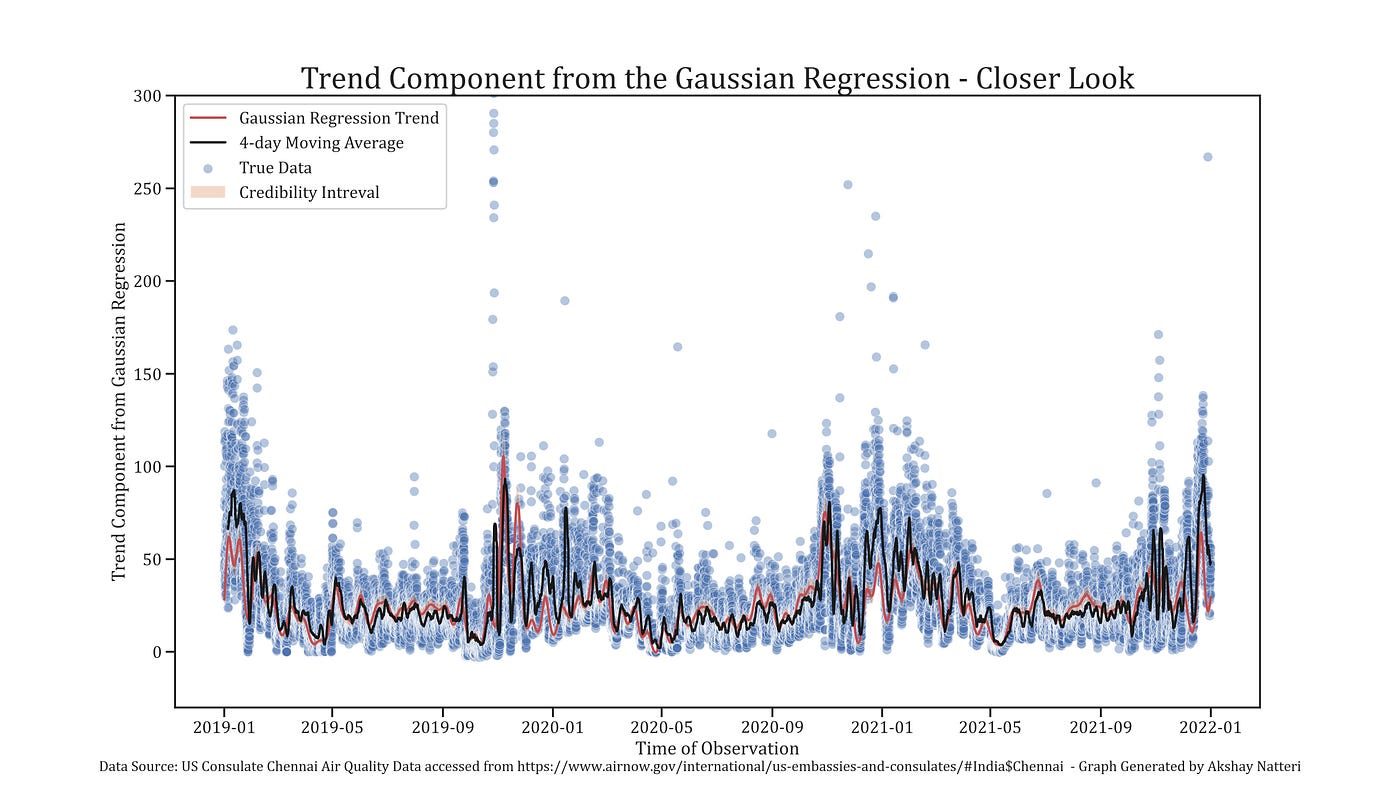Trend Component of the Gaussian Process Regression plotted along with the 4-day moving average (i.e., 96 hours). The y-axis of the graph is limited to 300, to show a clearer picture of the trend.

Hence, we can loosely assume that the ‘trend’ component is just the 4-day moving average of the data.

# The Special Day’s Component

Next, we look at the special days component defined by,

‘Beta j’ represents the coefficient corresponding to the indicator variable for special day ‘j’ (e.g., Deepavali, Bhogi, New Year etc.)

We have,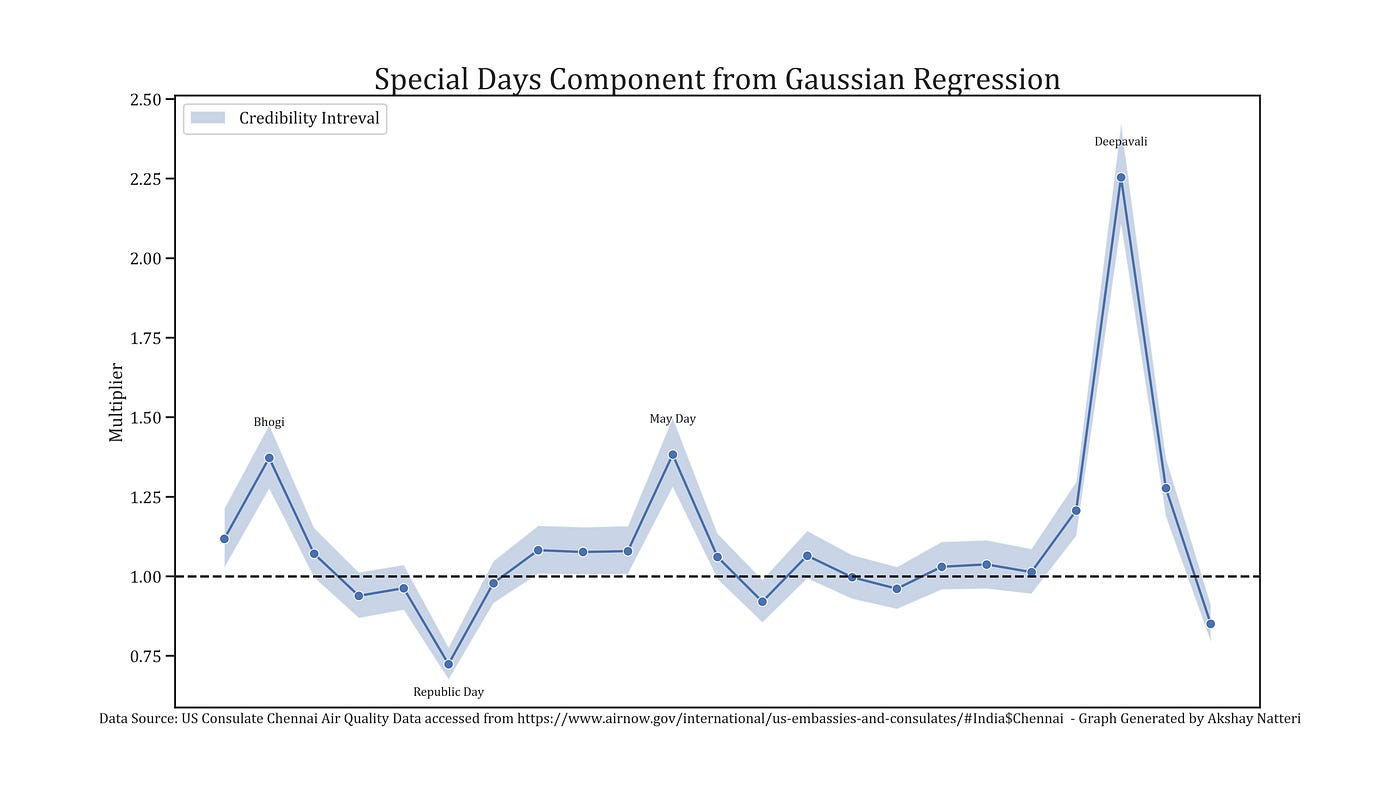The multiplicative effect of the special days coefficients is represented in this graph.

Deepavali, Bhogi, and May Day form peaks and Republic day forms the trough, just as in the previous results. However, this does not quite give us a complete picture. The absolute impact of the multiplier for the special days is contingent on the what it multiplies. For example, if the month component is large, even a small special day component could have a substantial amplification effect in absolute terms.

Say the month component is 100, then a special days multiplier of 2 would have an absolute effect of 200. However, if the month component is just 10, then a special days multiplier of even 10 (5 times larger than the previous case), would only have an absolute effect of 100 (half the absolute effect in the previous case). This makes comparing the values of the multipliers across the various special days difficult.

In order to get a better picture, I look at the aggregated (average) impact of the month and special day component, that is,

Since a special day, say Deepavali, could fall either in October or November depending on the year, I take the average of the aggregate of month and special day component across the three years. m_j(t) is a function that provides the month in which special day ‘j’ occurred during year ‘t’. The results are displayed in the figure below,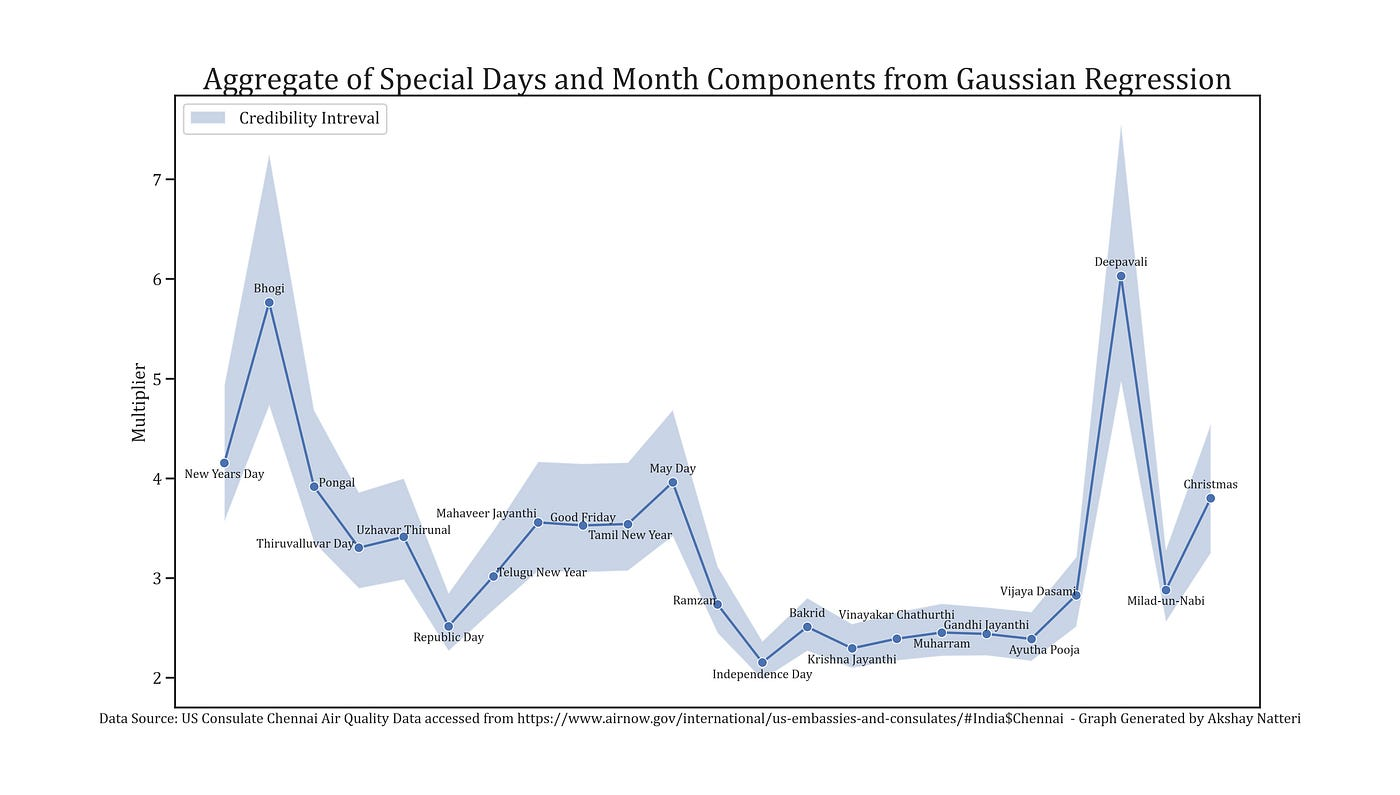The aggregate (average) effect of the month and special days component of the Gaussian Regression.

Once we take the month effects into consideration, we get a clearer picture. Loosely speaking, Bhogi and Deepavali multiply the prevailing average pollution levels by a factor of nearly 6.³ The other days are much lower as expected. Unlike the previous article, we are not in a position to make a judgement about the individual impact of the festivals in ‘absolute’ terms, but this provides us with a rough idea of the impact of the festivals. This obfuscation in the interpretation is the price we pay for a better fit.

Next, I plot the estimated mean from the Gaussian Regression, which is given by,

The results provides a much closer fit to the 4-day moving average (85.5% Pearson-correlation coefficient),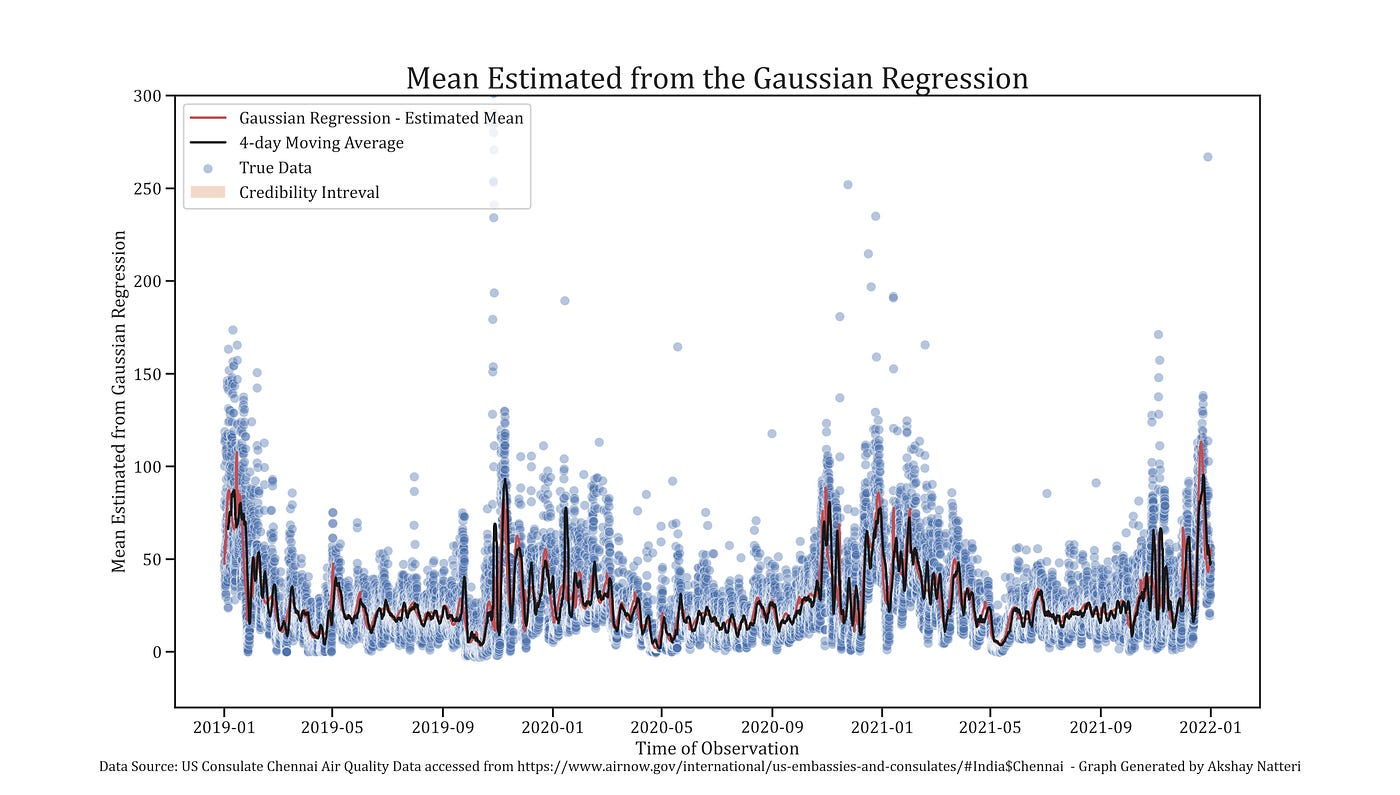Mean estimated from the Gaussian Process Regression plotted along with the 4-day moving average (i.e., 96 hours). The y-axis of the graph is limited to 300, to show a clearer picture of the trend.

It is important to note that the maximum value for the estimated mean from the Gaussian regression (which is the aggregate of the trend, month, and special days components) is 113 micrograms per cubic meter. This gives us a sense that in the period between 2019–2021, even the highest amplifying effect did not take the pollution to very unhealthy levels (on average). The reason for this is as stated in the previous article, prime of which are the extreme fluctuations in pollution level on special days owing to the lockdown, and the location of the monitoring stations.

# How Good a Fit is this Model?

The simple answer is a much, much better fit. The posterior predictive distribution of the current model is provided below,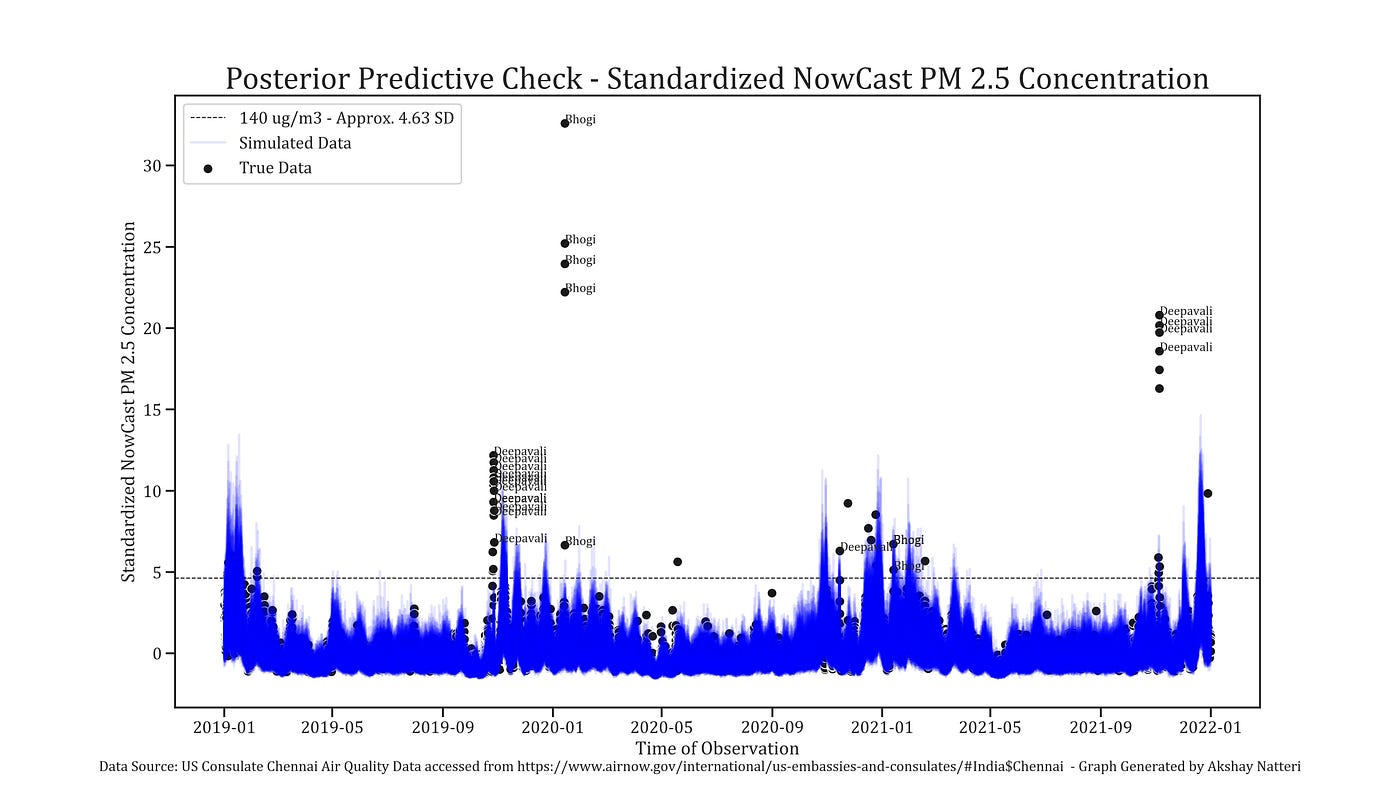Posterior predictive distribution of the Gaussian Process Models, 50 predictive-posterior realizations are plotted along with the actual hourly data.

In terms of distribution, it is quite a good fit⁴,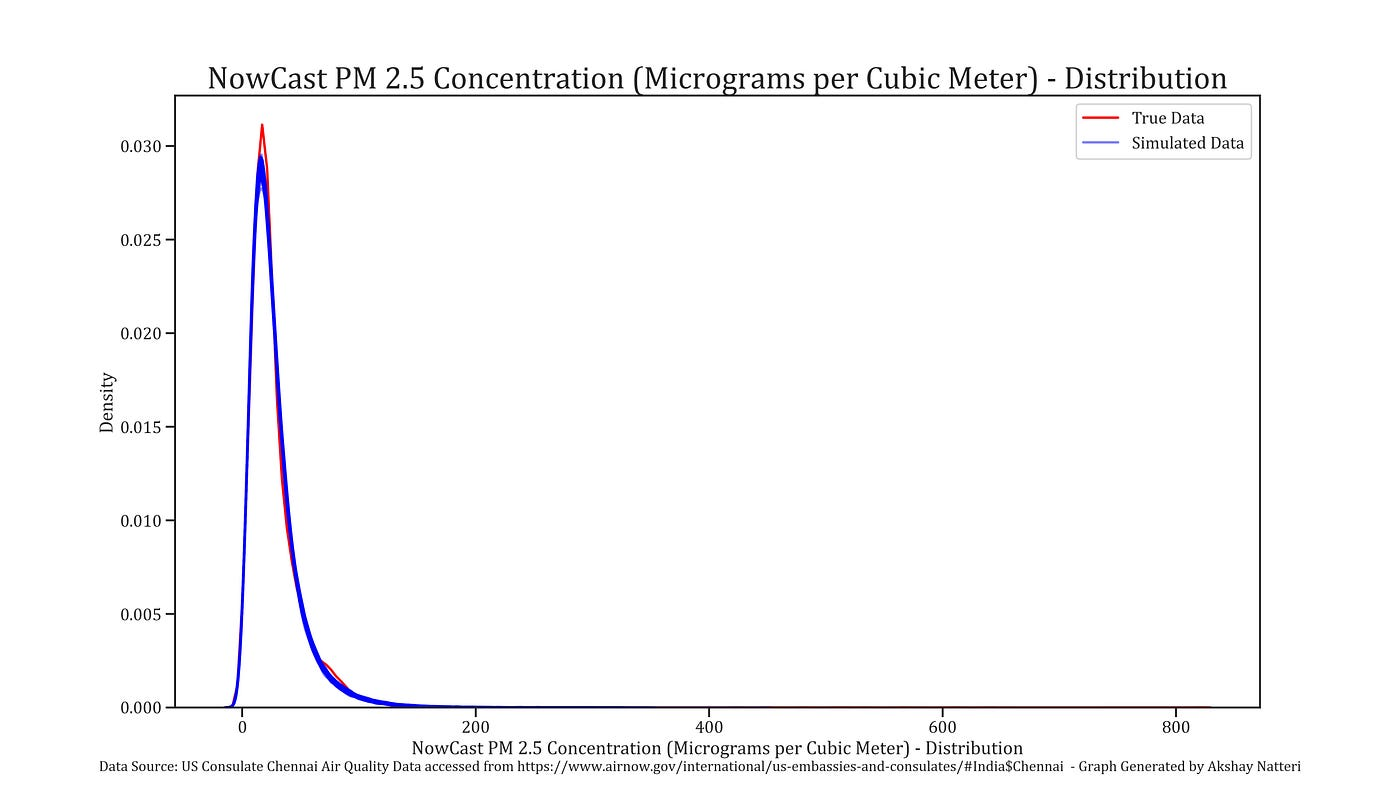Comparing the distribution of 100 predictive-posterior realizations with the true distribution of the data.

# Conclusion

The improved model tends to indicate that the pollution level during Bhogi and Deepavali is around 6 times the average prevailing pollution level during the time. This gives policy makers a rough estimate of expected pollution levels during such special festivals, to better guide the framing of rules and regulations.

# Footnotes

 The same approximation used in the previous article is used here. I use 200 basis functions, and a factor of 1.3 for determining the length of the compact set for computing the Eigen-functions and Eigen-values.

 I use a standard normal prior for the magnitude parameter of the SE kernel, and for Sigma (the standard deviation of the epsilon term). I use a normal prior with mean zero and standard deviation 0.1 for the length parameter in the SE kernel.

 It is important to note that the ‘trend’ component from the Gaussian process regression is substantially lower during Bhogi/Deepavali than the 4-day moving average. When the trend component is multiplied with the special day and month components it resembles the 4-day moving average. Hence, the trend component loosely provides the counterfactual level of pollution in the absence of the special day (I refer to this as prevailing average pollution level in the text of the article) .

 The simulated data tends to slightly over-predict the PM 2.5 concentration.

--

--

--

Applied Econometrician

Love podcasts or audiobooks? Learn on the go with our new app.

## Generating fake data with pandas, very quickly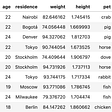## 7 Technical Concepts Every Data Science Beginner Should Know## How to Handle Categorical Features## Working with Open Data## How To Find A Data Science Internship In 2020## TIME SERIES FORECASTING AND ANALYSIS : ARIMA AND SEASONAL-ARIMA## Instacart Market basket analysis case study.## LSTMs for Music Generation## Akshay Natteri

Applied Econometrician

## Neat R Plots with the Cairo Graphics Library## Example of time series with Stata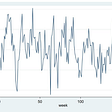## Drug-disease association prediction## Process of creating the Random Time Series using R Programming